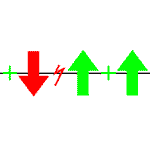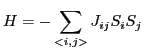Computing Exact Ground States of Ising Spin GlassesA spin-glass instance in the Ising model is given by n spins that can either point up or down. Spin i and j are coupled with coupling strength Jij. We mainly consider systems in which spins are located on the sites of a d-dimensional lattice with nearest neighbor interactions. The Hamiltonian energy function H is given bywhere the sum runs over all coupled spins. For a spin i pointing up, its spin variable Si takes value Si=1, otherwise Si=-1. A ground state is a spin configuration that attains the global minimum of the energy function H. In contrast to heuristic algorithms used by many physicists, we determine provably exact ground states. Calculating an exact ground state of a spin glass in the Ising model amounts to determining a maximum cut in the associated graph of interactions. The algorithm has been developed at the Computer Science Department in Cologne in collaboration with several other researchers. It is quite effective in practice and makes it possible to gain further insight into the ruling physics of the systems.